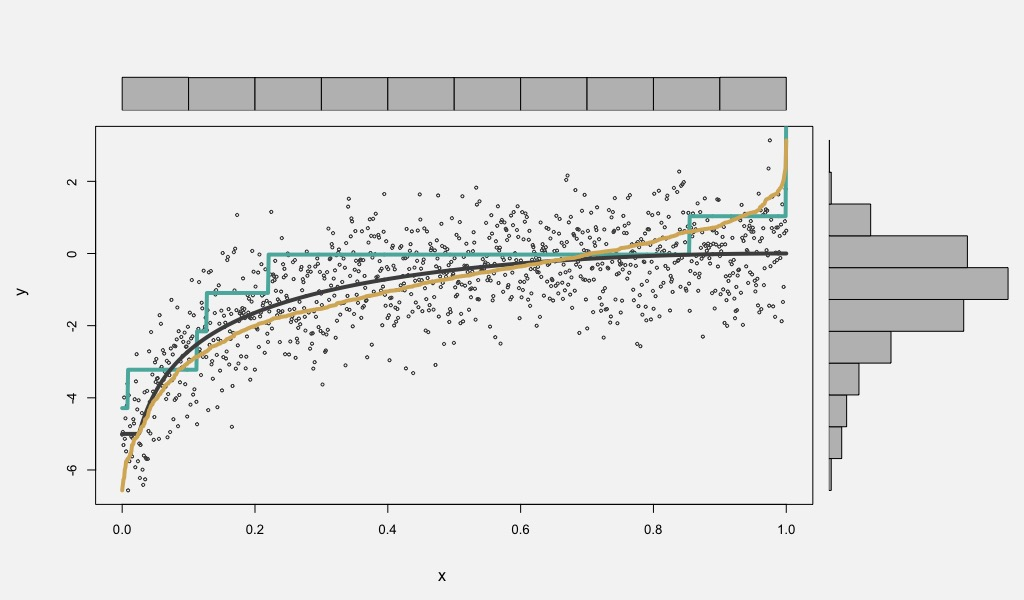# Implementing minimum-Wasserstein deconvolutionRecently, Rigollet and Weed (2019)1 introduced a consistent estimator for the problem of uncoupled isotonic regression. The authors also proposed a computationally efficient procedure for computing a relaxed version of the estimator, which achieves the same minimax rate.

Rigollet and Weed’s estimator relaxed estimator minimizes the Wasserstein-2 distance between the discrete, uniform measure on the observed data $${y_1, \dots, y_n}$$ – named $$\hat{\pi}$$ – and a quantization of the pushforward of the discrete uniform measure on the design points $${x_1, \dots, x_n}$$ under the unknown regression function $$f$$ convolved with a noise distribution $$\mathcal{D}$$.

To implement Rigollet and Weed’s relaxed minimum-$$W_2$$ estimator, one solves

\begin{aligned} \hat{\mu} \in \text{argmin}_{\mu \in \mathcal{M}_{\mathcal{A}, V}} W_2^2 \left(\Pi_{\mathcal{A}\sharp}(\mu \star \mathcal{D}), \hat{\pi} \right) \end{aligned}

where $$\Pi_{\mathcal{A}\sharp}(\mu \star \mathcal{D})$$ is the pushforward of the measure $$\mu \star \mathcal{D}$$ by the projection operator defined in Rigollet and Weed (2019). This operator discretizes the continuous measure $$\mu \star \mathcal{D}$$, concentrating its mass on an equi-spaced partition of the interval $$[-V, V]$$.

Because both measures considered are discrete, finding the Wasserstein distance between a given $$\Pi_{\mathcal{A}\sharp}(\mu \star \mathcal{D})$$ and $$\hat{\pi}$$ is equivalent to solving the linear program

\begin{aligned} \underset{\gamma_{ij}}{\text{minimize}}~~\sum_{ij} \gamma_{ij} &(y_i - \alpha_j)^2 \\ \text{subject to}~~\sum_{i} \gamma_{ij} &= a_j &j = 0, \dots, N \\ \sum_{j} \gamma_{ij} &= \hat{\pi}_i &i = 1, \dots, n \\ \gamma_{ij} &\geq 0 \end{aligned}

where $$\alpha_j$$ is the $$j^{\text{th}}$$ element in the partition of $$[-V, V]$$ defined in Rigollet and Weed (2019), $$y_i$$ is the $$i^{\text{th}}$$ observed data point, and $$a_j = \Pi_{\mathcal{A}\sharp}(\mu \star \mathcal{D})_j$$, the amount of probability mass placed at $$\alpha_j$$.

From the dual problem to this linear program, we can obtain the subgradient of the $$W_2^2$$ distance with respect to the measure $$a$$. This subgradient is equal to the vector of optimal dual solutions corresponding to the first equality constraint in the linear program above. Therefore,

\begin{aligned} \frac{\partial W_2^2}{\partial a_j} = g_j^*~~~~~~~~j = 0, \dots, N \\ \end{aligned}

where $$g_j^\ast$$ is the $$j^{\text{th}}$$ component of the optimal dual vector.

Define $$\mu_i := \mu (\alpha_i)$$; in other words the measure weight under $$\mu$$ given to $$\alpha_i$$ (note that this is different than $$a_j$$, which is the measure weight under $$\Pi_{\mathcal{A}\sharp}(\mu \star \mathcal{D})$$). Then based on the definition of the projection operator $$\Pi_{\mathcal{A}\sharp}$$, $$a_j$$ varies with $$\mu_i$$ according to the relationship

\begin{aligned} a_j (\mu_1, \dots, \mu_N) = \sum_{i=1}^N \mu_i \int_{\alpha_j}^{\alpha_{j+1}} p(x ; \alpha_j, \sigma^2) dx \end{aligned}

where $$p$$ is the density function of the noise distribution $$\mathcal{D}$$. Hence, the partial derivatives of the $$a$$’s with respect to each $$\mu_i$$ are

\begin{aligned} \frac{\partial a_j}{\partial \mu_i} = \int_{\alpha_j}^{\alpha_{j+1}} p(x ; \alpha_j, \sigma^2) dx \end{aligned}

The Chain Rule then yields subgradients of the $$W_2^2$$ objective with respect to the measure weights $$\mu_1, \dots, \mu_N$$ according to

\begin{aligned} \frac{\partial W_2^2}{\partial \mu_i} = \sum_{j} \frac{\partial W_2^2}{\partial a_j} \frac{\partial a_j}{\partial \mu_i} \end{aligned}

With these subgradients in hand, a projected descent algorithm of the form

\begin{aligned} \mu_{t+1} = \text{Proj}_\mathcal{S} \left( \mu_t - \lambda_t \frac{d W_2^2}{d \mu} \right) \end{aligned}

may be used to arrive at the optimal value $$\mu^*$$.

To speed convergence to the optimal $$\mu^*$$, the CFM step-size rule2 can be used to determine the value of $$\lambda_t$$ at each iteration. The operator $$\text{Proj}_\mathcal{S}$$ is the projection operator onto the probability simplex.

An R implementation of the minimum-Wasserstein estimator can be found at https://github.com/j-g-b/uncoupled.

1 Rigollet, P., Weed, J. (2019) Uncoupled isotonic regression via minimum Wasserstein deconvolution, Information and Inference: A Journal of the IMA.

2 Camerini P.M., Fratta L., Maffioli F. (1975) On improving relaxation methods by modified gradient techniques. In: Balinski M.L., Wolfe P. (eds) Nondifferentiable Optimization. Mathematical Programming Studies, vol 3. Springer, Berlin, Heidelberg.